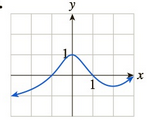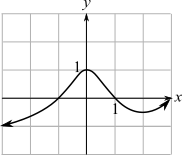Chapter 10.5, Problem 30EFinite Mathematics and Applied Cal...

7th Edition
Stefan Waner + 1 other
ISBN: 9781337274203

Solutions

Chapter
SectionFinite Mathematics and Applied Cal...

7th Edition
Stefan Waner + 1 other
ISBN: 9781337274203
Textbook Problem

In Exercises 27–30, find the approximate coordinates of all points (if any) where the slope of the tangent is (a) 0, (b) 1, and (c) − 1 . [HINT: See Quick Example 3.](a)

To determine

The coordinates on the graph at which the slope of tangent 0 from the provided graph is,Explanation

Given information:

The provided graph is:

The slope of the tangent line is given by the rate of change or derivative on the graph of the function f at the particular point x=a.

The tangent line in the graph of function f at the point P(a,f(a)) which is a straight line passing through point P with slope f(a).

From the graph it can be observed that the curve is first increases up to x=0, then starts decreasing and then again start increasing at x=2

(b)

To determine

The coordinates on the graph at which the slope of tangent one from the provided graph is,(c)

To determine

The coordinates on the graph at which the slope of tangent 1 from the provided graph is,Still sussing out bartleby?

Check out a sample textbook solution.

See a sample solution

The Solution to Your Study Problems

Bartleby provides explanations to thousands of textbook problems written by our experts, many with advanced degrees!

Get Started

Evaluate limx01+tanx1+sinxx3.

Calculus: Early Transcendentals

In Exercises 311, find the derivative of the function. f(t)=t3+2t

Calculus: An Applied Approach (MindTap Course List)

In Exercises 2730, determine all values of x for which each function is discontinuous. 30. f(x) = |2x|x

Applied Calculus for the Managerial, Life, and Social Sciences: A Brief Approach